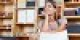# Basics of Bernoulli’s Principle

Bernoulli’s principle states that the total mechanical energy of the fluid which is moving comprises of the gravitational potential energy of elevation, energy-related to fluid pressure, and kinetic energy of the fluid motion, remains constant.

Bernoulli’s principle was formulated by Daniel Bernoulli and the Bernoulli equation was derived by Leonhard Euler in the year 1752. Daniel Bernoulli was a Swiss mathematician and physicist who published Hydrodynamica in the year 1738. His work was mainly focused on explaining the speed of the fluid and its effect on pressure. Along with Bernoulli’s principle, Daniel also contributed to the study of the kinetic theory of gases.

Bernoulli’s equation is related to the conservation of energy principle and is applied to fluids that are in motion. As the definition of Bernoulli’s principle states, Bernoulli’s equation is related to pressure, kinetic energy, and gravitational potential energy. Also, there were a few assumptions that were made about the fluid and its properties such as density, viscosity, etc, so that the derivation of Bernoulli’s equation becomes easy.

Assumptions related to Bernoulli’s principle

• The flow is steady and incompressible
• A non-viscous fluid is used with a constant density
• The flow is along a single streamline

Bernoulli Equation

Following is the Bernoulli equation:

P + 1/2ρv2 + ρgh = constant

Where,

• P is the pressure
• ρ is the density of the fluid
• v is the velocity of the fluid
• h is the height of the container from which the fluid is flowing
• g is the gravitational potential energy

Applications of Bernoulli Principle

Following are the applications of the Bernoulli principle:

• The spinning of a ball: A stationary ball is said to exhibit a symmetric flow of airstream. Also, when a ball is thrown by giving a spin, there will be a curve and that’s because one of the sides of the ball experiences less pressure and this is explained by Bernoulli principle.
• Wings of the aeroplane: This is one of the best applications of the Bernoulli principle. The wing is also designed in a unique way. The wing is tilted upward at a small angle and the upper surface is long so that the air moves faster over the wing. The net upward lift is created by reducing the pressure on the upper side of the wing.

Interested to learn more about the derivation of Bernoulli’s equation and other Physics related concepts such as stress-strain curve, visit BYJU’S.